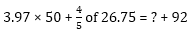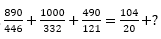SBI Clerk Quantitative Aptitude Quiz

With the increasing level in exams, quantitative aptitude has become the ineluctable hitch. Generally, questions asked in this section are calculative and lengthy that consumes your time. This subject can do wonders if you always keep a check on your accuracy, speed and time. Accuracy is what matters the most. To help you prepare the section we have provided a well-defined SBI Clerk Main 2019 study plan. You can also Prepare from the study notes to clear your basic concepts. Attempt this quantitative aptitude quiz and check your performance for the upcoming SBI Clerk exam 2019. Following is the quiz of 4th August, that inculcate the important topics from Line Di, Miscellaneous And Quadratic Equations.

Directions (1-2): In the given questions, two quantities are given, one as ‘Quantity I’ and another as ‘Quantity II’. You have to determine relationship between two quantities and choose the appropriate option (compare only magnitudes)

Q1. Quantity I- ‘Area of rectangle’ Sum of circumference of circle and perimeter of rectangle is 220 cm and area of circle is 1386 sq. cm. If length of rectangle is   more than radius of circle.
Quantity II- ‘Perimeter of square’  Circumference of the circle is 132 cm and area of circle is 710 sq. cm more than area of square
Quantity I > Quantity II
Quantity I < Quantity II
Quantity I ≥ Quantity II
Quantity I ≤ Quantity II
Quantity I = Quantity II or no relation

Q2. Quantity I – Veer takes two times more than the time taken by Sameer to complete a work. Sameer and veer started to work alternatively starting with Sameer on first day and Veer on second day. If both completed work in 36 days, then find in how many days Sameer and Veer will complete the work together, if both work with their double efficiency?
Quantity II – Tap P can fill a swimming pool in 8 days and tap Q can fill the same swimming pool in 24 days, while tap R can empty the swimming pool in 16 days. If all three taps are opened together in swimming pool, then in how much time swimming pool will be filled completely?
Quantity I > Quantity II
Quantity I < Quantity II
Quantity I ≥ Quantity II
Quantity I ≤ Quantity II
Quantity I = Quantity II or no relation

Directions (3-5): The following questions are accompanied by three statements (A), (B), and (C). You have to determine which statement(s) is/are sufficient /necessary to answer the questions.

Q3. What is the length of train ‘A’?
A. Train A takes 8 second to cross a pole
B. Train A cross train B coming from opposite side in 8 second, speed of train B is 25 m/sec and length of train B is half of that of train A.
C. Train A takes 10 sec to cross two poles 100 m apart.
Only A and B together
Only A and C together
Any two of them
Either B alone or A and C together
All statements are required

Q4. In how many days B and C together can complete the work?
A. Per day efficiency of A, B and C is in the ratio 3 : 2 : 4
B. A and B together can complete the work in 7.2 days
C. Time taken by A, B and C alone to complete the work is in the ratio of 4 : 6 : 3.
Only A and B together
Either A and B or B and C
Any two of them
Either B alone or A and C together
All statements are required

Q5. What is the base radius of the cone?
A. Total surface area of the cone is 462 cm²
B.and height = 7√3 cm
Only A and B together
Only A and C together
Any two of them
Either B alone or A and C together
All statements are required

Q6. Satish started from point A in a boat to reach point B. After 6.5 hours he covered only 20% of the distance and reach at point M. Now, Satish started from point M and reached at mid point of A and B and came back to M in 29.25 hours. In what time Satish can cover the distance between B and A if he started from B?
58.5 hours
32.5 hours
65 hours
62.5 hours
40 hours

Q7. The distance between 2 stations x and y is 650 km. If two trains(A and B) start together at the same time from both stations towards each other and meet after 10 hrs but if train A has started 4 hrs 20 min after the train B then they meet after 8 hours. Find the speed of trains.
35 km/hr, 30 km/hr
35 km/hr, 40 km/hr
25 km/hr, 40 km/hr
20 km/hr, 45 km/hr
32.5 km/hr, 32.5 km/hr

Q8. A pedestrian and a cyclist start simultaneously towards each other from Aurangabad and Paithan which are 40 km apart and meet 2 hours after the start. Then they resume their trips and the cyclist arrives at Aurangabad 7 hours 30 minutes earlier than the pedestrian arrives at Paithan. Which of these could be the speed of the pedestrian?
4 km/hr
5 km/hr
3 km/hr
6 km/hr
7 km/hr

Q9. A 180 m long train crosses another 270 m long train running in the opposite direction in 10.8 seconds. If the shorter train crosses a pole in 12 seconds, what is the speed of longer train?
98 km/hr
96 km/hr
90 km/hr
88 km/hr
None of these

Q10. A boat while travelling upstream covers a distance of 18 km at the speed of 3 km/h, whereas while travelling downstream it covers the same distance at a speed of 9 km/h. What is the speed of the boat in still water?
3 km/h
5 km/h
7 km/h
Cannot be determined
None of these

Directions (11-15): What approximate value will come in place of (?)

Q11. 697 × 3 + 898 × 4 – 999 × 5 = ?
555
700
656
858
650

Q12.128
148
152
186
118

Q13.1.5
9
6
4
7

Q14.52
72
42
58
62

Q15. 4.19 × 3.92 × (19.79 ÷ 2.97) ÷ 4.92 × 65.89 = ?
2430
2556
2616
2716
1408